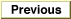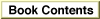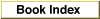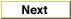# Legacy DocumentImportant: The information in this document is obsolete and should not be used for new development.

Inside Macintosh: QuickDraw GX Environment and Utilities /
Chapter 8 - QuickDraw GX Mathematics / QuickDraw GX Mathematics Reference
Mathematical Functions / Fixed-Point Operations

### FractMultiply

You can use the `FractMultiply` function to calculate the product of two numbers.

```fract FractMultiply (fract multiplicand, fract multiplier);
```
`multiplicand`
The number to be multiplied by the multiplier.
`multiplier`

The number by which the multiplicand is to multiplied.
function result
The product of two numbers.
##### DESCRIPTION
The `FractMultiply` function calculates the product of two numbers, specified in the `multiplicand` and `multiplier` parameters. If the parameters are a and b, the product a b is returned.

The format of the number returned depends on the respective number formats of the `multiplicand` and `multiplier` parameters. The operation has a bias of 30 bits; in general, the bias of the resulting number is the sum of the biases of the input numbers, shifted right by 30 bits. Thus if either the `multiplicand` or `multiplier` parameter is `fract`, then the result is the same fixed-point format as the other argument.

Table 8-5 shows the bias of the FractMultiply result. The dashed line indicates that the resulting bias is not equivalent to long, fixed, or fract. Use the rules of the operation to determine it
`FractMultiply` result bias
longfixedfract
long------long
fixed------fixed
fractlongfixedfract

##### SPECIAL CONSIDERATIONS
`FractMultiply` does not pin its result in the case of an overflow; the result returned is modulo 4.